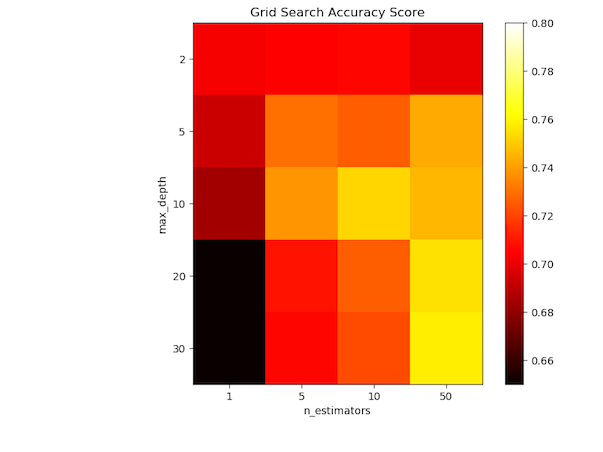# Number of trees and estimators

Random forests are an ensemble over a large number of decision trees. The number of trees used is controlled by a parameter called n_estimators. Below you can see a heatmap of the accuracy of a random forest classifier. Different values of maximum depth (max_depth) are shown on the vertical axis. Different numbers of estimators (n_estimators) are shown on the horizontal axis. How does the performance of the classifier depend on these two hyperparameters in this case?